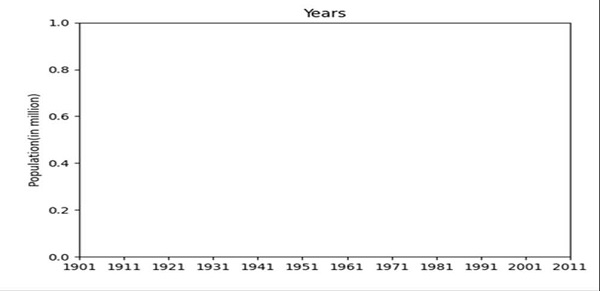# How to get a matplotlib Axes instance to plot to?

To get the axes instance, we will use the subplots() method.

## Steps

• Make a list of years.

• Make a list of populations in that year.

• Get the number of labels using np.arrange(len(years)) method.

• Set the width of the bars.

• Create fig and ax variables using subplots() method, where default nrows and ncols are 1.

• Set the Y-axis label of the figure using set_ylabel().

• Set the title of the figure, using set_title() method.

• Set the x-ticks with x that is created in step 3, using set_xticks method.

• Set the xtick_labels with years data, using set_xticklabels method.

• Use plt.show() method to show the figure.

## Example

from matplotlib import pyplot as plt
import numpy as np

years = [1901, 1911, 1921, 1931, 1941, 1951, 1961, 1971, 1981, 1991, 2001, 2011]
population = [237.4, 238.4, 252.09, 251.31, 278.98, 318.66, 361.09, 439.23, 548.16, 683.33, 846.42, 1028.74]

x = np.arange(len(years))       # the label locations
width = 0.35                    # the width of the bars

fig, ax = plt.subplots()        # axes instance

ax.set_ylabel('Population(in million)')
ax.set_title('Years')
ax.set_xticks(x)
ax.set_xticklabels(years)

plt.show()

## Output